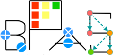Basic Polynomial Algebra Subprograms (BPAS)  v. 1.652
BPASRegularChain< Field, RecursiveFieldPoly > Class Template Referenceabstract

An abstract class defining the interface of a regular chain. More...

`#include <polynomial.h>`

Simplified semantic inheritance diagram for BPASRegularChain< Field, RecursiveFieldPoly >:Full inheritance diagram for BPASRegularChain< Field, RecursiveFieldPoly >:
[legend]

## Public Member Functions

virtual BPASRegularChain< Field, RecursiveFieldPoly > & operator= (const BPASRegularChain< Field, RecursiveFieldPoly > &)=0

virtual BPASRegularChain< Field, RecursiveFieldPoly > & operator= (BPASRegularChain< Field, RecursiveFieldPoly > &&)=0Public Member Functions inherited from BPASTriangularSet< Field, RecursiveFieldPoly >
virtual BPASTriangularSet< Field, RecursiveFieldPoly > & operator= (const BPASTriangularSet< Field, RecursiveFieldPoly > &)=0

virtual BPASTriangularSet< Field, RecursiveFieldPoly > & operator= (BPASTriangularSet< Field, RecursiveFieldPoly > &&)=0

virtual int numberOfVariables () const =0

virtual std::vector< Symbolvariables () const =0

virtual RecursiveFieldPoly select (const Symbol &) const =0

virtual void lower (const Symbol &, BPASTriangularSet< Field, RecursiveFieldPoly > &) const =0

virtual void upper (const Symbol &, BPASTriangularSet< Field, RecursiveFieldPoly > &) const =0

virtual RecursiveFieldPoly pseudoDivide (const RecursiveFieldPoly &, std::vector< RecursiveFieldPoly > *, RecursiveFieldPoly *) const =0

virtual RecursiveFieldPoly normalForm (const RecursiveFieldPoly &, std::vector< RecursiveFieldPoly > *) const =0

## Detailed Description

### template<class Field, class RecursiveFieldPoly> class BPASRegularChain< Field, RecursiveFieldPoly >

An abstract class defining the interface of a regular chain.